# Peer review 1. International Reputasi, International, Procceding.

Sunarto, Andang Peer review 1. International Reputasi, International, Procceding. [Dataset]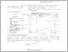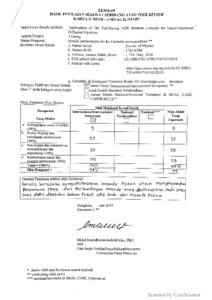Preview
Text
application of the full sweep AOR iteration concept for space fractional diffusion equation.pdf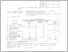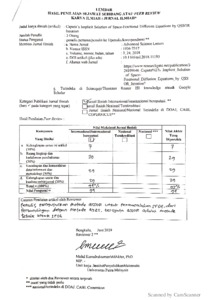Preview
Text
caputo's implicit solution of space-fractional diffusion equations by QSSOR iteration.pdf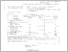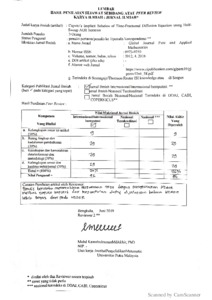Preview
Text
caputo's implict solution of time-fractional diffusion equation using Half-sweep AOR iteration.pdf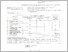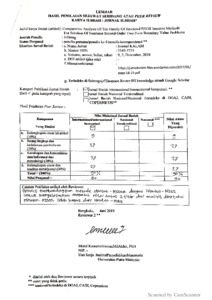Preview
Text
comparative analysis of the family of newton HSSOR iterative methods for solution of nonlinier second order two point boundry value problems.pdf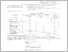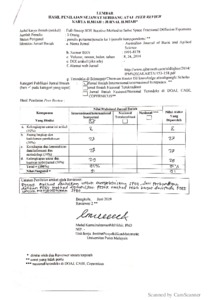Preview
Text
full-sweep SOR iterative method to solve space fractional diffusion equations.pdf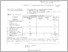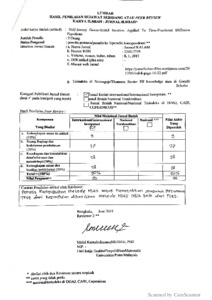Preview
Text
half sweep gauss seidel iteration applied to time fractional diffusion equations.pdf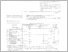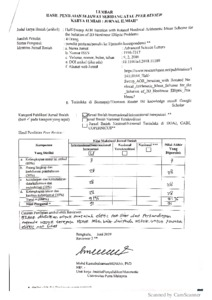Preview
Text
half-sweep AOR iteration with rotated nonlocal arithmetic mean scheme for the solution of 2D nonlinier elliptic problems.pdf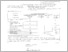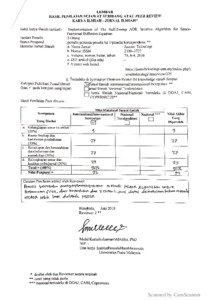Preview
Text
implementation of the half sweep AOR iterative algorithm for space fractional diffusion equation.pdf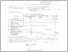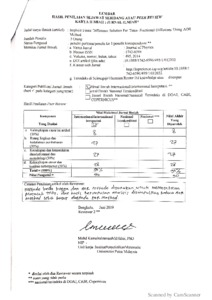Preview
Text
implicit finite difference solution for time-fractional diffusions using AOR method.pdf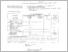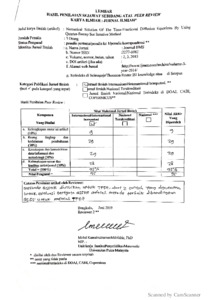Preview
Text
nunmerical solution of the time-fractional diffusion equations by using quarter-sweep sor iterative method.pdf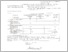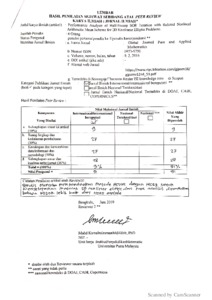Preview
Text
performance analysis of Half-Sweep SOR iteration with rolated nonlocal arithmetic mean scheme for 2D nonlinier elliptic problems.pdf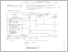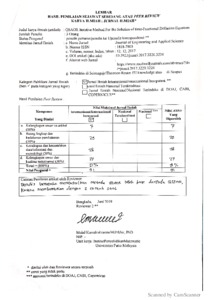Preview
Text
QSAOR iterative method for the solution of time fractional diffusion equation.pdf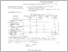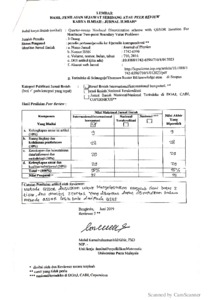Preview
Text
quarter sweep nonlocal discretization scheme with QSSOR iteration for nonlinier two point boundary value problems.pdf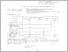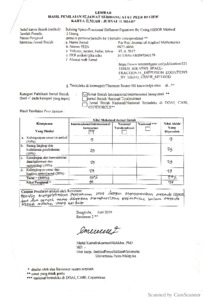Preview
Text
solving space fractional diffusion equations by using HSSOR Method.pdf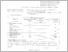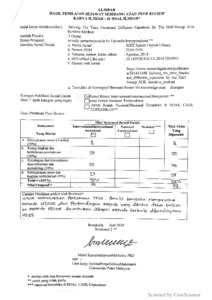Preview
Text
solving the time fractional diffusion equations by the Half-Sweep SOR iterative Method.pdf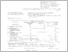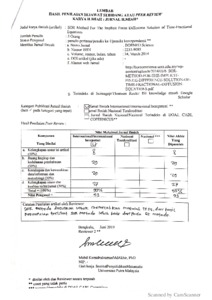Preview
Text
SOR Method for the implicit finite difference solution of time-fractional equations.pdf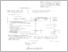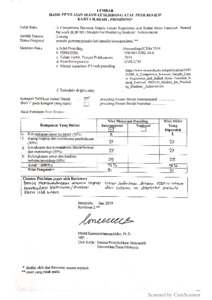Preview
Text
A comparison between simple linear regression and radial basis function neural network (RBF NN) models for predicting students' Achievement.pdf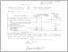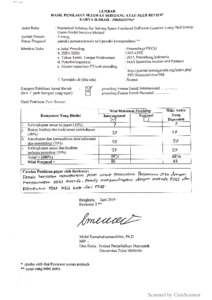Preview
Text
numerical solution for solving space-fractional diffusion equation using half-sweep gauss-seidel iterative method.pdf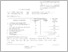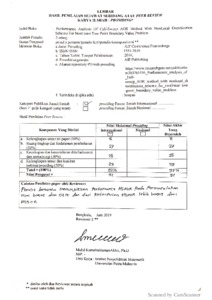Preview
Text
performance analysis of Half-Sweep AOR Method with nonlocal dicretization scheme for nonlinier two-point boundry value problem.pdfView Item